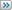# Help solving a programming challenge optimally

Printable View

• September 2nd, 2018, 05:55 PM
programming_challeng
Help solving a programming challenge optimally
Here is the problem:
You are given an array of digits 0-9 and an integer *n*. The array may contain duplicates of any given digit. Find all the integers which can be formed by contatenating digits from the input array and are less than *n*. Digits from the input array can be repeated in an element in the output.

For example, given as inputs [2, 5, 8] and *n* = 223, then the following should be the output:

[2, 5, 8, 22, 25, 28, 52, 55, 58, 82, 85, 88, 222]

Obviously any integer with fewer digits than *n* would be accepted and any with more denied.
But how to most efficiently find those with the same number of digits?A solution I know of would be to have nested for loops, one for each digit in the input integer, and form every possible combination of digits from the input array and check it against *n*. This seems clunky though and I'm wondering if there's a better way.
• September 3rd, 2018, 04:23 AM
wolle
Re: Help solving a programming challenge optimally
Quote:

Originally Posted by programming_challengA solution I know of would be to have nested for loops, one for each digit in the input integer, and form every possible combination of digits from the input array and check it against *n*. This seems clunky though and I'm wondering if there's a better way.

You can use the branch-and-bound approach. It's optimal in the sense that only valid numbers are generated (as opposed to generating all numbers and discarding invalid ones)

With your example, think of a tree where every node has three children, each holding a number. At the first node level the numbers are 200, 500 and 800, at the second level 20, 50 and 80 and the leaf level 2, 5 and 8. So below the root there will be 3 node levels with 3, 3*3 and 3*3*3 nodes respectively, that is 39 in total.

The tree is traversed in depth-first order. An accumulator is incremented with the node numbers when going down the tree and decremented when going up. The accumulator will always hold the sum of all nodes from root to current node.

If the accumulator holds a sum < N when a leaf node is reached that's a valid number. If the accumulator holds a sum >= N at a non-leaf node that's an invalid number and there's no reason to continue farther down the tree. It's because this cannot turn the number valid again and this is the bound that allows whole branches to be cut away so a full tree search may be avoided. The suggested order of the numbers in the tree matters because it allows for the largest branch-cuts.

It's not necessary to explicitly generate the tree (with pointers and nodes and stuff), it can be traversed implicitly. Several implementations are possible including a modified version of the nested loops approach you thought of initially. The "clunkiness" can be avoided by replacing the fixed nested loops with recursive calling of one loop to the wanted nesting depth.
• September 21st, 2018, 01:50 AM
wolle
Re: Help solving a programming challenge optimally
Here's a "proof of concept" I wrote in preparation for my previous post,

Code:

```void test() {         const int N = 900;         int acc = 0;         for (const int n1 : {200, 500, 800}) {                 if ((acc + n1) >= N) break; // bound found - cut branch                 acc += n1;                 for (const int n2 : {20, 50, 80}) {                         if ((acc + n2) >= N) break; // bound found - cut branch                         acc += n2;                         for (const int n3 : {2, 5, 8}) {                                 if ((acc + n3) >= N) break; // bound found - cut branch                                 acc += n3;                                 std::cout << acc << std::endl;                                 acc -= n3;                         }                         acc -= n2;                 }                 acc -= n1;         } }```
It's a direct implementation of the OPs example in C++. It's clear from the code structure that it lends itself to a recursive implementation.